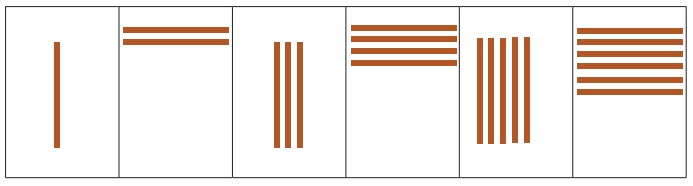Home | | Maths 2nd Std | Patterns in numbers

# Patterns in numbers

Keywords: Rule, Arrange, Order

UNIT 2

Patterns

Patterns in numbers

Recall

Observe and complete the patterns in numbers.Keywords: Rule, Arrange, Order

Travel Through

Roll and Scroll game

* Two students can do the activity.

* Make a number board with numbers from 1 to 37 as shown in the picture.

* The first player throws a dice and a number appears on the face of the dice say 2.

* The other player places a coin on the number 2 and skips to number position in twos such as 4,6,8,10 and so on till the last number. The first player records each of the number positions.* Now, the second player gets the chance to throw the dice. When the number 2 appears again the player tells all the number positions jumped orally. If he gets a number 3, then the first player moves a coin by skip counting in threes.

* The second player record each of the number positions jumped. The above procedure is continued till the players are familiarised with the number patterns starting from 2, 3, 4, 5 and 6.

Learn

Find the rule and complete the patterns.Activity

Count and write the number of ice sticks and complete the patterns using the rules.Practice

Find the rule and write them in each of the pattern given below.Pleasure Time

1) Write the number patterns for the given instructions.

i) The last number is 21. The first number is 13. Write the rule.13  (+2)  15 (+2)17  (+2)  19  (+2) 21

ii) The pattern begins with 39. The next number is 3 less than the previous number.39(−3), 36(−3), 33(−3), 30(−3) 27

iii) The middle number in the pattern is 45. The number previous to it is 40.35 (+5), 40 (+5), 45 (+5), 50 (+5), 55

2) Draw sticks to continue the pattern.Try This

Draw the missing black keys in a pattern.Tags : Patterns | Term 3 Chapter 2 | 2nd Maths , 2nd Maths : Term 3 Unit 2 : Patterns
Study Material, Lecturing Notes, Assignment, Reference, Wiki description explanation, brief detail
2nd Maths : Term 3 Unit 2 : Patterns : Patterns in numbers | Patterns | Term 3 Chapter 2 | 2nd Maths